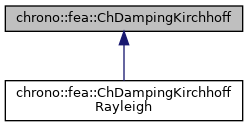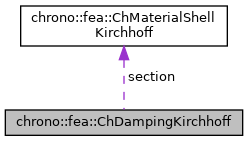chrono::fea::ChDampingKirchhoff Class Referenceabstract

## Description

Base interface for damping of thin shells (Kirchoff-Love shell theory, without shear effects) to be used in a ChMaterialShellKirchhoff.

Children classes should implement a ComputeStress function that returns generalized stresses given time derivatives of strains as: {n,m}=f({e',k'})

#include <ChMaterialShellKirchhoff.h>

Inheritance diagram for chrono::fea::ChDampingKirchhoff:[legend]
Collaboration diagram for chrono::fea::ChDampingKirchhoff:[legend]

## Public Member Functions

virtual void ComputeStress (ChVector<> &n, ChVector<> &m, const ChVector<> &deps, const ChVector<> &dkur, const double z_inf, const double z_sup, const double angle)=0
Compute the generalized cut force and cut torque, caused by structural damping, given actual deformation speed and curvature speed. More...

virtual void ComputeDampingMatrix (ChMatrixRef R, const ChVector<> &deps, const ChVector<> &dkur, const double z_inf, const double z_sup, const double angle)
Compute the 6x6 tangent material damping matrix, ie the jacobian [Rm]=dstress/dstrainspeed. More...

## Public Attributes

ChMaterialShellKirchhoffsection

## ◆ ComputeDampingMatrix()

 void chrono::fea::ChDampingKirchhoff::ComputeDampingMatrix ( ChMatrixRef R, const ChVector<> & deps, const ChVector<> & dkur, const double z_inf, const double z_sup, const double angle )
virtual

Compute the 6x6 tangent material damping matrix, ie the jacobian [Rm]=dstress/dstrainspeed.

This must be overridden by subclasses if an analytical solution is known (preferred for high performance), otherwise the base behaviour here is to compute [Rm] by numerical differentiation calling ComputeStress() multiple times.

Parameters
 R 6x6 material damping matrix values here deps time derivative of strains de_11/dt, de_22/dt, de_12/dt dkur time derivative of curvature dk_11/dt, dk_22/dt, dk_12/dt z_inf layer lower z value (along thickness coord) z_sup layer upper z value (along thickness coord) angle layer angle respect to x (if needed) -not used in this, isotropic

Reimplemented in chrono::fea::ChDampingKirchhoffRayleigh.

## ◆ ComputeStress()

 virtual void chrono::fea::ChDampingKirchhoff::ComputeStress ( ChVector<> & n, ChVector<> & m, const ChVector<> & deps, const ChVector<> & dkur, const double z_inf, const double z_sup, const double angle )
pure virtual

Compute the generalized cut force and cut torque, caused by structural damping, given actual deformation speed and curvature speed.

This MUST be implemented by subclasses.

Parameters
 n forces n_11, n_22, n_12 (per unit length) m torques m_11, m_22, m_12 (per unit length) deps time derivative of strains de_11/dt, de_22/dt, de_12/dt dkur time derivative of curvature dk_11/dt, dk_22/dt, dk_12/dt z_inf layer lower z value (along thickness coord) z_sup layer upper z value (along thickness coord) angle layer angle respect to x (if needed)

Implemented in chrono::fea::ChDampingKirchhoffRayleigh.

The documentation for this class was generated from the following files:
• /builds/uwsbel/chrono/src/chrono/fea/ChMaterialShellKirchhoff.h
• /builds/uwsbel/chrono/src/chrono/fea/ChMaterialShellKirchhoff.cpp